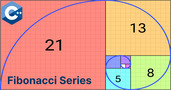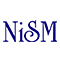Fibonacci Number

# Fibonacci Number

clickHere
Updated on Jul 26, 2023 18:05 IST

In this article, we will discuss Fibonacci numbers, it’s application and how to generate them using Python, Java and C.

## Introduction

In this article, we will discuss the Fibonacci Number in Python, Java and C.

In mathematics, we have different types of numbers like integer, natural, real, rational etc. along with these, we have a beautiful number with the remarkable property known as a Fibonacci number.

The Fibonacci number was discovered by Italian mathematician Lionardo Pisano (aka Fibonacci) in the thirteen century.

Now, let’s understand the Fibonacci numbers in detail.

## What is the Fibonacci number?

In simple term, Fibonacci numbers are the sequence of number in which each number is a sum of previous two numbers.

First few Fibonacci numbers are:Fibonacci Series Program in C++
The Fibonacci series is an intriguing mathematical idea that is popular for practicing in Python, especially for those just starting out.Fibonacci Series in Python
Fibonacci Series is a sequence of numbers where each number is the sum of the two previous numbers. This article will discuss the Fibonacci Series, How to Create a Fibonacci...read moreFibonacci Program in C
The Fibonacci Series is a sequence of numbers where each number is a sum of the previous two numbers. This article will explore how to implement the Fibonacci Series in...read more

## Application of Fibonacci Number

Fibonacci numbers has a wide variety of applications from nature, human body, music to the stock market.

• Nature
• Plants show Fibonacci number on the arrangement of their leaves and seeds on flower heads
• Human Body
• Length of the bone in hand are in Fibonacci number
• Stock Market
• Trader use “Fibonacci Retracements Level”, it allows traders to compare a high point to a low point. These level often include 23.6%, 38.2%, 61.8%, and 78.6%.

## Fibonacci Number in Python

We will generate Fibonacci numbers in Python by two methods:

• Loop

Firstly, input the number of values (positive integer), we want to generate the sequence, and then initialize the first and second value.

Now, we will use the for loop to iterate the number from 0 to user-defined and finally using the print we will produce the result.

```#Generate the Fibonacci Number using Loop

num = int(input("Enter the number"))  #enter the number of terms needed

#set first two fibonacci numbers

F_0 = 0
F_1 = 1

for n in range(0, num):  #Febonacci number is defined only for positive integer
if(n<=1):
F_n = n  # this will only return the first two fibonacci numbers
else:
F_n = F_0 + F_1  #every fibonacci number is sum of previous two
F_0 = F_1
F_1 = F_n
print(F_n)```
• Recursion

Firstly, we will define a function (feb (n)) and then use if else condition:

• if n = 0, return 0 (first fibonacci number)
• elif n = 1, return 1 (second fibonacci number)
• else return feb(n-1) + feb(n-2) (i.e. a recursive function used to calculate the nth term of the sequence)

Finally, we will use to iterate and calculate each term recursively using the input from the user.

```#Generate the Fibonacci number using recursion
#define a recursive function

def feb(n):
if n == 0:
return 0  #first fibonacci number F_0 = 0
elif n == 1:
return 1  #second fibonacci number F_1 = 0
else:
return (feb(n - 1) + feb(n - 2))  #every fibonacci number is sum of previous two

n = int(input("Enter the number"))  #enter the number of terms needed
for i in range(0, n):
print (feb(i))```

Must Check: Python Online Course & Certification

Must Check: What is Python?

Must Check: Python Projects for BeginnersFor Loop in Python (Practice Problem) – Python Tutorial – Shiksha Online
for loop in python is used to iterate over a sequence or an iterable object (such as a list, tuple, or string). In this article, we will discuss 18 different...read moreTutorial – for Loop in PythonConditional Statements in Python – Python Tutorial
A conditional statement as the name suggests itself, is used to handle conditions in your program. These statements guide the program while making decisions based on the conditions encountered by...read more

## Fibonacci Number in JAVA

Firstly, we will intialize the program with first two digit of the Fibonacci Series i.e. 0 and 1 respectively. Then we use FOR loop:

• Print the First term (F_0)
• Print the next term (F_n = F_0 + F_1)

Then, finally assign the value of second term to first term and next term to second term.

```// Generate the Fibonacci Sequence using JAVA

class Main {
public static void main(String[] args) {

int n = 10, F_0 = 0, F_1 = 1;
System.out.println("Fibonacci Sequence of first" + n + " terms are:");

for (int i = 1; i <= n; ++i) {
System.out.print(F_0 + ", ");

// compute the next term
int F_n = F_0 + F_1;
F_0 = F_1;
F_1 = F_n;
}
}
}
```

Must Check: Top Java Online Course & Certifications

Must Check: What is Java?

## Fibonacci Number in C

Firstly, we will initialize the first two terms (F_0 and F_1), then use the for loop to print the next n terms of Fibonacci series.

```#include <stdio.h>
int main() {

int i, n;

// Set first two Fibonacci number
int F_0 = 0, F_1 = 1;

// initialize the next term
int F_n = F_0 + F_1;

// enter the number of terms needed
printf("Enter the number of terms: ");
scanf("%d", &n);

// print the next term
for (int i = 0; i <= n; ++i) {
printf("%d, ", F_n);
F_0 = F_1;
F_1 = F_n;
F_n = F_0 + F_1;
}

return 0;
}
```

Must Check: C Programming Online Course & Certification

Must Check: What is C programming?

## Conclusion

In this article, we have discussed how to generate the Fibonacci number in Python, Java and C.

## FAQs

What is Fibonacci Series?

Fibonacci numbers are the sequence of numbers defined by the linear equation, F(n) = F(n-1) + F(n-2), for n = 3, 4, ... and F(0) = 0, F(1) = F(2) = 1.

What are the first 10 Fibonacci Numbers in the Fibonacci Series?

The first 10 Fibonacci numbers are, 0, 1, 1, 2, 3, 5, 8, 13, 21, 34, 55.

What is the use of Fibonacci Series?

Fibonacci Series is used in Finance (Stock Market Analysis), Computer Science (Efficient Search), Music ( Tuning Musical Instruments), Art (Creating Pleasing Visual Design).

clickHere

Vikram has a Postgraduate degree in Applied Mathematics, with a keen interest in Data Science and Machine Learning. He has experience of 2+ years in content creation in Mathematics, Statistics, Data Science, and Mac... Read Full Bio

## Trending Data Science CoursesData Science with Python
UDEMY4.2Become a Data Science Professional with IIT Certification in Advanced ProgrammingBecome a Data Science Professional with IIT Certif...
Guvi5.0Become a Data Science Professional with IIT Certification in Advanced ProgrammingBecome a Data Science Professional with IIT Certif...
Guvi5.0Starts 15 Dec

## Top Picks & New Arrivals